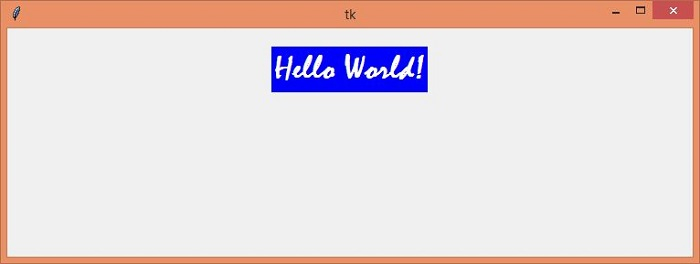What is the difference between a variable and StringVar() of Tkinter?

TkinterServer Side ProgrammingProgramming

A variable in Tkinter is used to store the values of any data. For a Tkinter application, we can store the values in two ways −

• by defining the value programmatically, or

• by storing the value through user Input.

A normal variable can be used to set the value for any application whenever it is required. However, we can take the user input by creating an instance of the StringVar() object. When we specify a Tkinter variable such as textvariable, for a widget (textvariable = myvar), the widget automatically gets updated whenever the value of the variable changes. However, there might be times when we want to modify the value of the widget through the variable.

Example

In this program, we will update the Label Widget from the StringVar(value). Whenever we want to update the value of Tkinter StringVar(), we have to change its value.

#Import the required Libraries
from tkinter import *
from tkinter import ttk
#Create an instance of tkinter frame
win = Tk()

#Set the geometry of tkinter frame
win.geometry("750x250")

#Create a StringVar to accept user input
var= StringVar(value= "Hello World!")

#Create a Label
label= Label(win,textvariable=var, font= ('Mistral 28 bold'), background= 'blue', foreground="white")